## Analysis of Propellers

A relatively simple method of predicting the performance of a propeller (as well as fans or windmills) is the use of Blade Element Theory. In this method the propeller is divided into a number of independent sections along the length. At each section a force balance is applied involving 2D section lift and drag with the thrust and torque produced by the section. At the same time a balance of axial and angular momentum is applied. This produces a set of non-linear equations that can be solved by iteration for each blade section. The resulting values of section thrust and torque can be summed to predict the overall performance of the propeller.

The theory does not include secondary effects such as 3-D flow velocities induced on the propeller by the shed tip vortex or radial components of flow induced by angular acceleration due to the rotation of the propeller. In comparison with real propeller results this theory will over-predict thrust and under-predict torque with a resulting increase in theoretical efficiency of 5% to 10% over measured performance. Some of the flow assumptions made also breakdown for extreme conditions when the flow on the blade becomes stalled or there is a significant proportion of the propeller blade in windmilling configuration while other parts are still thrust producing.

The theory has been found very useful for comparative studies such as optimising blade pitch setting for a given cruise speed or in determining the optimum blade solidity for a propeller. Given the above limitations it is still the best tool available for getting good first order predictions of thrust, torque and efficiency for propellers under a large range of operating conditions.

A propeller blade can be subdivided as shown into a discrete number of sections.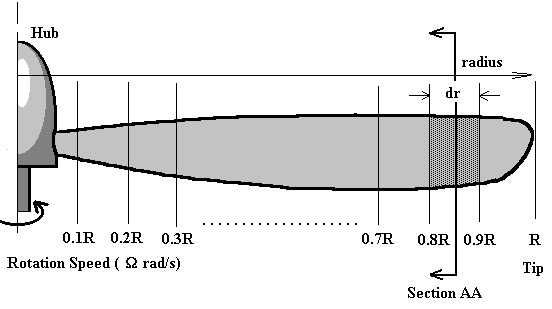For each section the flow can be analysed independently if the assumption is made that for each there are only axial and angular velocity components and that the induced flow input from other sections is negligible. Thus at section AA (radius = r) shown above, the flow on the blade would consist of the following components.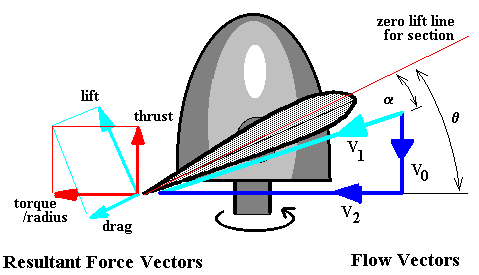V0 -- axial flow at propeller disk, V2 -- Angular flow velocity vector

V1 -- section local flow velocity vector, summation of vectors V0 and V2

Since the propeller blade will be set at a given geometric pitch angle (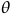) the local velocity vector will create a flow angle of attack on the section. Lift and drag of the section can be calculated using standard 2-D aerofoil properties. (Note: change of reference line from chord to zero lift line). The lift and drag components normal to and parallel to the propeller disk can be calculated so that the contribution to thrust and torque of the compete propeller from this single element can be found.

The difference in angle between thrust and lift directions is defined as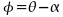The elemental thrust and torque of this blade element can thus be written as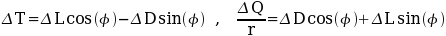Substituting section data (CL and CD for the given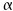) leads to the following equations.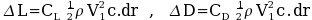per blade

where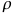is the air density, c is the blade chord so that the lift producing area of the blade element is c.dr.

If the number of propeller blades is (B) then,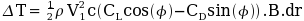. . . . . . . . .(1)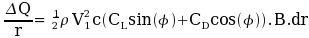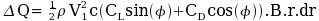. . . . . . . . .(2)

2. Inflow Factors

A major complexity in applying this theory arises when trying to determine the magnitude of the two flow components V0 and V2. V0 is roughly equal to the aircraft's forward velocity (Vinf) but is increased by the propeller's own induced axial flow into a slipstream. V2 is roughly equal to the blade section's angular speed (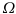r) but is reduced slightly due to the swirling nature of the flow induced by the propeller. To calculate V0 and V2 accurately both axial and angular momentum balances must be applied to predict the induced flow effects on a given blade element. As shown in the following diagram the induced flow components can be defined as factors increasing or decreasing the major flow components.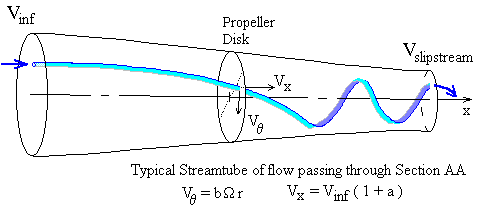So for the velocities V0 and V2 as shown in the previous section flow diagram,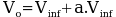where a -- axial inflow factor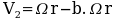where b -- angular inflow factor (swirl factor)

The local flow velocity and the angle of attack for the blade section is thus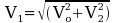. . . . . . . . . . . . . . . . . . . . . . (3)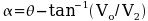. . . . . . . . . . . . . . . . . . . . . . .(4)

3. Axial and Angular Flow Conservation of Momentum

The governing principle of conservation of flow momentum can be applied for both axial and circumferential directions.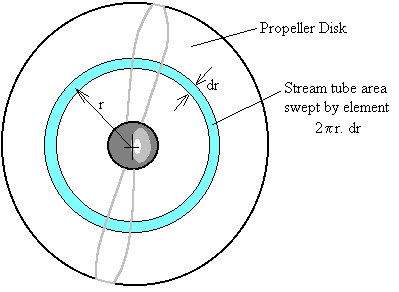For the axial direction, the change in flow momentum along a stream-tube starting upstream, passing through the propeller at section AA and then moving off into the slipstream, must equal the thrust produced by this element of the blade.

To remove the unsteady effects due to the propeller's rotation, the stream-tube used is one covering the complete area of the propeller disk swept out by the blade element and all variables are assumed to be time averaged values.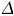T = change in momentum flow rate

= mass flow rate in tube x change in velocity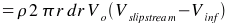By applying Bernoulli's equation and conservation of momentum, for the three separate components of the tube, from freestream to face of disk, from rear of disk to slipstream far downstream and balancing pressure and area versus thrust, it can be shown that the axial velocity at the disk will be the average of the freestream and slipstream velocities.

V0 = (Vinf + Vslipstream)/2, that means Vslipstream = Vinf ( 1 + 2a)

Thus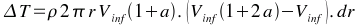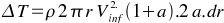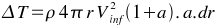. . . . . . . . (5)

For angular momentumQ = change in angular momentum rate for flow x radius

= mass flow rate in tube x change in circumferential velocity x radius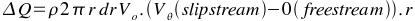By considering conservation of angular momentum in conjunction with the axial velocity change, it can be shown that the angular velocity in the slipstream will be twice the value at the propeller disk.Thus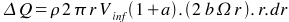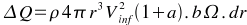. . . . . . . . . . . . . (6)

Because these final forms of the momentum equation balance still contain the variables for element thrust and torque, they cannot be used directly to solve for inflow factors.

However there now exists a nonlinear system of equations (1),(2),(3),(4),(5) and (6) containing the four primary unknown variablesT,Q, a, b. So an iterative solution to this system is possible.

4. Iterative Solution procedure for Blade Element Theory.

The method of solution for the blade element flow will be to start with some initial guess of inflow factors (a) and (b). Use these to find the flow angle on the blade (equations (3),(4)), then use blade section properties to estimate the element thrust and torque (equations (1),(2)). With these approximate values of thrust and torque equations (5) and (6) can be used to give improved estimates of the inflow factors (a) and (b). This process can be repeated until values for (a) and (b) have converged to within a specified tolerance.

It should be noted that convergence for this nonlinear system of equations is not guaranteed. It is usually a simple matter of applying some convergence enhancing techniques (ie Crank-Nicholson under-relaxation) to get a result when linear aerofoil section properties are used. When non-linear properties are used, ie including stall effects, then obtaining convergence will be significantly more difficult.

For the final values of inflow factor (a) and (b) an accurate prediction of element thrust and torque will be obtained from equations (1) and (2).

5. Propeller Thrust and Torque Coefficients and Efficiency.

The overall propeller thrust and torque will be obtained by summing the results of all the radial blade element values.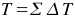(for all elements), and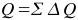(for all elements)

The non-dimensional thrust and torque coefficients can then be calculated along with the advance ratio at which they have been calculated.

CT = T/(n2D4) and CQ = Q/(n2D5) for J = Vinf/(nD)

where n is the rotation speed of propeller in revs per second and D is the propeller diameter.

The efficiency of the propeller under these flight conditions will then be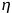(propeller) = J/(2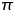).(CT/CQ).

6. Software Implementation of Blade Element Theory

Two programming versions of this propeller analysis technique are available. The first is a demonstration program which can be used to calculate thrust and torque coefficients and efficiency for a relatively simple propeller design using standard linearised aerofoil section data. The blade is assumed to have a constant pitch (p) so that the variation ofwith radius is calculated from the standard pitch equation.

p = 2r tan().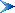Return to Contents Page